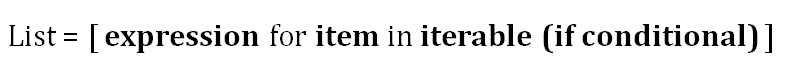# Crystal Clear Explanation of Python List ComprehensionsA highly intuitive way for understanding them .Crystal Clear Explanation of Python List Comprehensions

List comprehension is a way of creating lists based on other iterables such as sets, tuples, other lists, and so on. It can also be described as representing for and if loops with a simpler and more appealing syntax. List comprehensions are relatively faster than for loops though.

The basic structure of a list comprehension is as follows.(image by author)

It seems quite simple but might get tricky in some cases. In this article, we will start from a very simple list comprehension and steadily increase the complexity. I will clearly explain how to represent and understand highly complex list comprehensions as well.

List comprehensions are preferred over for and if loops in most cases because

• They are relatively faster than for loops.
• They are considered to be more pythonic than for loop and map function.
• The syntax of list comprehension is easier to read and understand.

Let’s start with a simple example.

``words = ['data','science','machine','learning']``

We want to create a list that contains of the length of each word in the words list. Let’s perform the task using both a for loop and list comprehension.

``````#for loop
a = []
for word in words:
a.append(len(word))
#list comprehension
b = [len(word) for word in words]
print(f"a is {a}")
print(f"b is {b}")
a is [4, 7, 7, 8]
b is [4, 7, 7, 8]``````

## How I'd Learn Data Science If I Were To Start All Over Again

A couple of days ago I started thinking if I had to start learning machine learning and data science all over again where would I start?

## Data Science With Python Training | Python Data Science Course | Intellipaat

🔵 Intellipaat Data Science with Python course: https://intellipaat.com/python-for-data-science-training/In this Data Science With Python Training video, you...

## Data Science Projects | Data Science | Machine Learning | Python

Practice your skills in Data Science with Python, by learning and then trying all these hands-on, interactive projects, that I have posted for you.

## Data Science Projects | Data Science | Machine Learning | Python

Practice your skills in Data Science with Python, by learning and then trying all these hands-on, interactive projects, that I have posted for you.

## Data Science Projects | Data Science | Machine Learning | Python

Practice your skills in Data Science with Python, by learning and then trying all these hands-on, interactive projects, that I have posted for you.